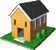Ontario Research Centre for Computer Algebra Technical Reports
The Ontario Research Centre for Computer Algebra

# The UWO ORCCA Reading Room

## UWO ORCCA TR-07-03 Summary

The solution to W exp W = A is not always LambertW(A) Robert M. Corless, Hui Ding, and David Jeffrey, Paper submitted to ISSAC 2007.

Abstract: The solutions of the matrix equation \$S\exp S = A\$ are studied. This is motivated by the study of systems of delay differential equations \$y'(t) = A y(t-1)\$, which occur in some models of practical interest, especially in mathematical biology. This paper concentrates on the distinction between \textsl{evaluating a matrix function} and \textsl{solving a matrix equation}. As is well-known in the theory of computing matrix functions, there can be difficult, exceptional cases. This paper examines some of these; specifically, it shows that the matrix Lambert \$W\$ function evaluated at the matrix \$A\$ does not represent all possible solutions of \$S\exp S = A\$.

 About ORCCA Members Events & SeminarsORCCA Home Research Activities Positions Reading Room Contact Info